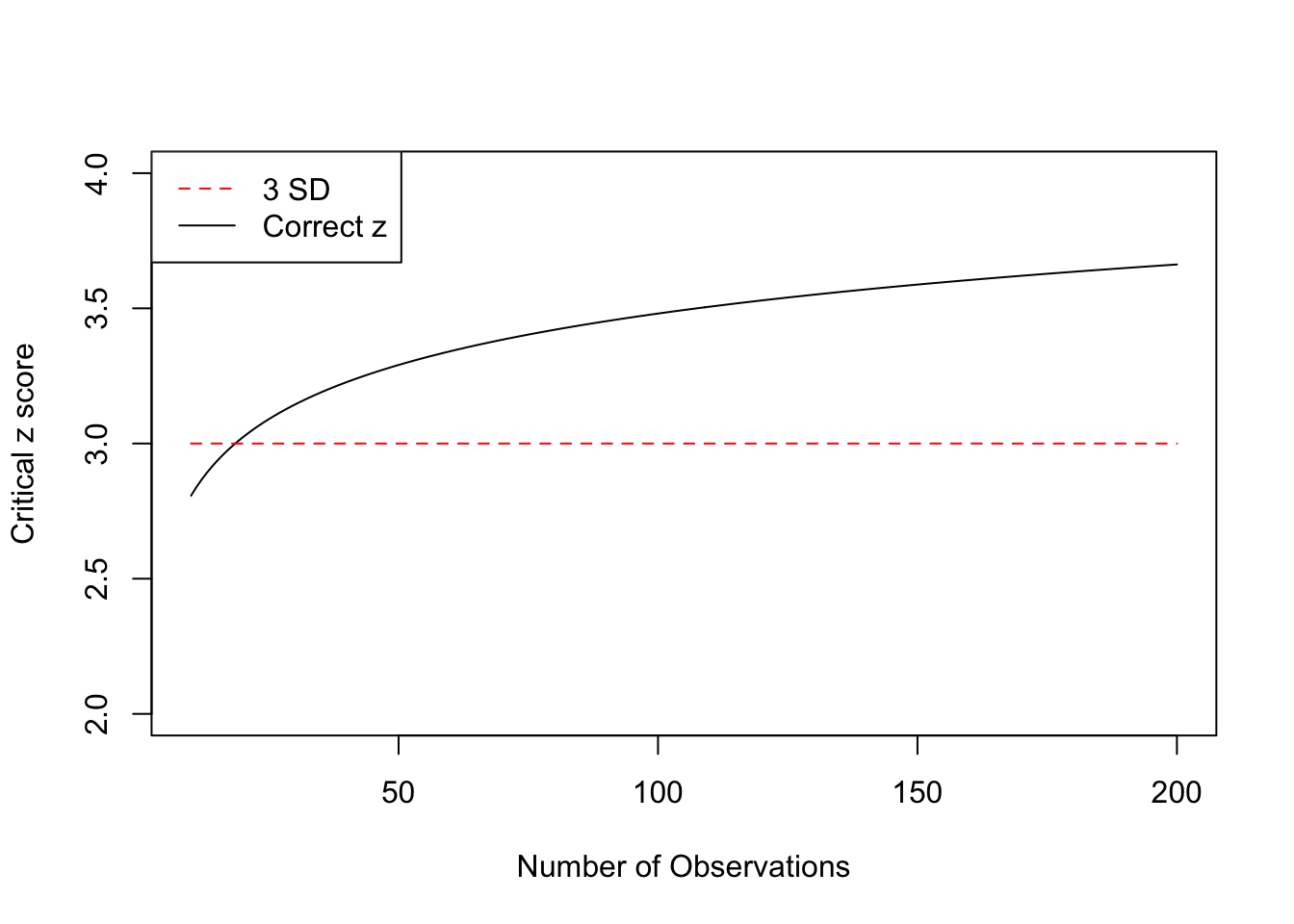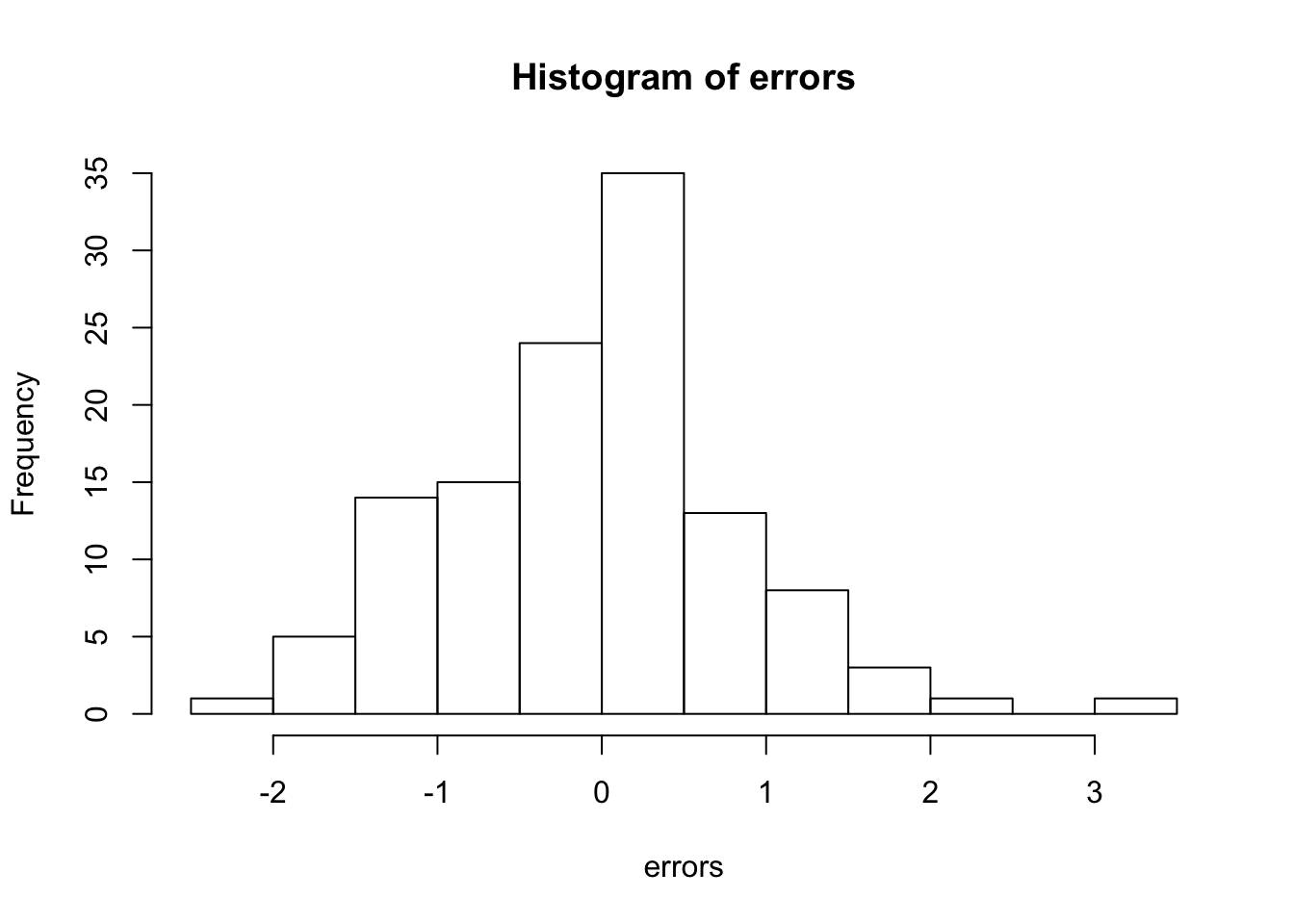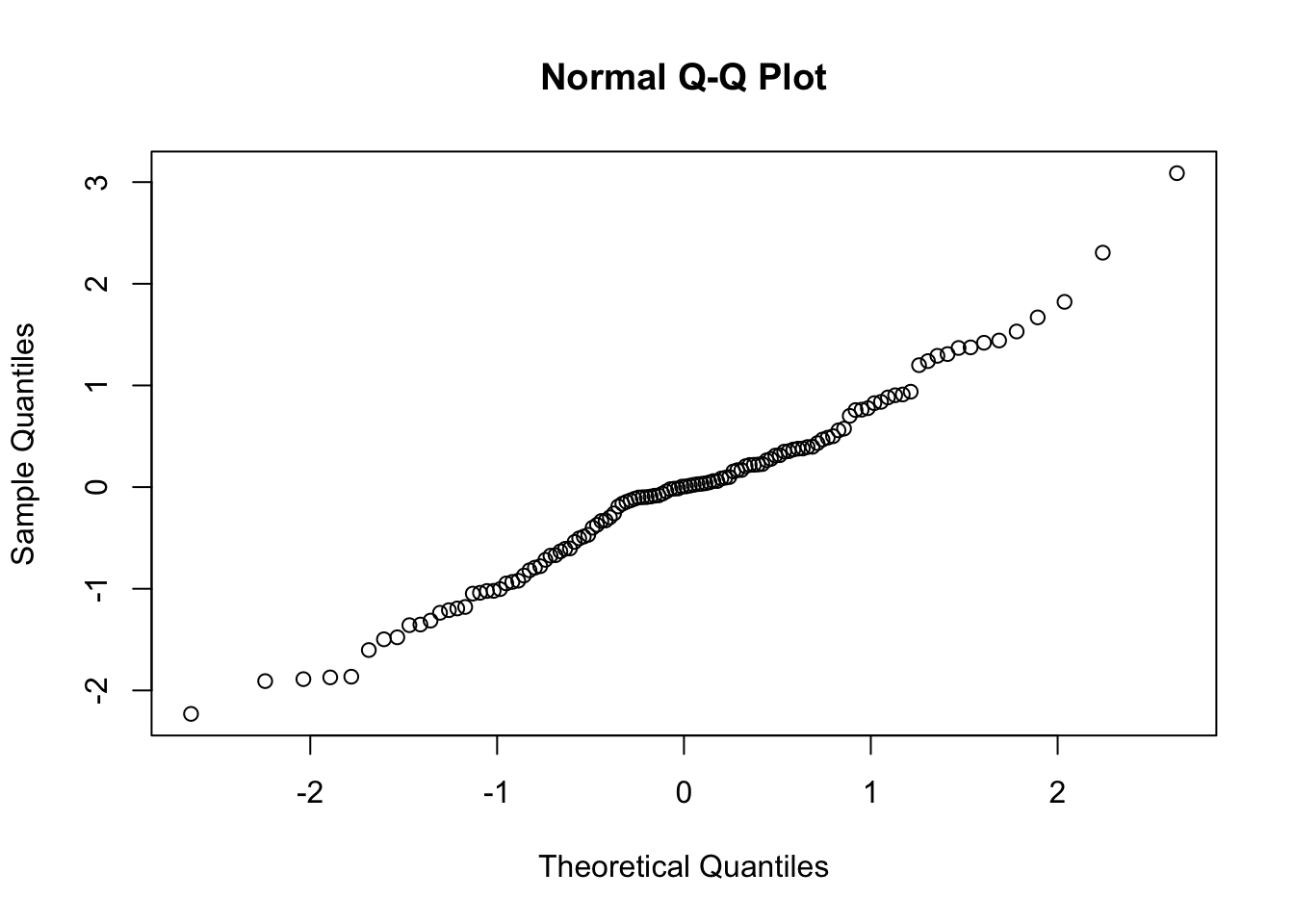# Outliers

Historically, set values based on standard deviations (2,3) or interquantile range (box-whisker) have been used as criteria for screening for outliers.

The preferred method for Recommendations is a Bonferri-corrected $$z$$-score method. First $$z$$-scores are calculated for each observation by taking $$z_{ij} = \epsilon_{ij}/s^2$$ where $$s^2$$ is the residual mean square. Then a critical $$z_{crit}$$ value is calculated from the normal distribution for a probability given by $$1-\frac{\alpha}{2n}$$ (commonly $$\alpha=0.05$$) and $$n=i \times j$$. Any $$y_{ij}$$ such that $$|z_{ij}|>z_{crit}$$ is marked as an outlier.

plot(10:200,qnorm(1-0.05/(2*(10:200))),type='l', ylim=c(2,4), lty=1,
xlab='Number of Observations',ylab='Critical z score')
segments(10,3,200,3,lty=2,col='red')
legend('topleft', legend=c("3 SD", "Correct z"),col=c("red", "black"),lty = c(2,1),lwd=1)## Rationale

Suppose we use $$\mu \pm 2\sigma$$ as the range of “normal” values, and consider any value outside that range as an outlier. The proportion of values drawn from a normal distribution falling between in that range is expected to be

pnorm(2) - pnorm(-2)
##  0.9544997

so, if we have $$n$$ greater than

ceiling(1/(1-(pnorm(2) - pnorm(-2))))
##  22

we would not be surprised to find an outlier using this criteria.

# Percent of observations between -3 and 3 std dev
(pnorm(3) - pnorm(-3))
##  0.9973002
#convert to a count (1/n)
ceiling(1/(1-(pnorm(3) - pnorm(-3))))
##  371

# Simulation

A variety trial with 30 varieties replicated 4 times has 120 plots. How many often would outliers be detected even when the errors are normally distributed?

## Single trial

We draw a random sample of errors from the $$z$$ distribution, and plot histogram and qqnorm plots

set.seed(200)
errors <- rnorm(120, 0,1)
hist(errors)qqnorm(errors)If we were to select outliers based on 3 sd, we would find at least one spurios outlier in this sample.

max(abs(errors))
##  3.087978

If we use a correct critical $$z$$, we would not identify outliers in this sample.

qnorm(1-0.05/(2*120))
##  3.529296

## Multiple Trials

Suppose we wish to combine 10 trials of 120 plots. How likely are we to identify spurious outliers?

trials <- 1:10
for(i in 1:10) {
errors <- rnorm(120, 0,1)
trials[i] <- max(abs(errors))
}
sum(trials>3)
##  5
sum(trials>qnorm(1-0.05/(2*120)))
##  2

At 3 sd, we find that 5 of the 10 trials have spurious outliers, but with adjusted $$z$$, we find only 2.

## Chauvenet’s Criterion

https://en.wikipedia.org/wiki/Chauvenet%27s_criterion